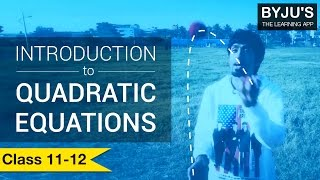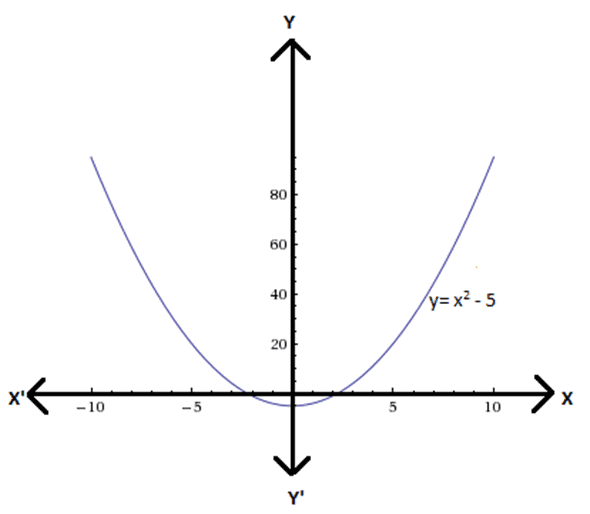The theory of equations deals with the solution of algebraic equations. Quadratic Equations are a particular case of the theory of equations. As it is known that quadratics are represented by parabolas which look similar to a smiley in layman terms. The motion of the balls while juggling, the motion of roller coaster in Disneyland all represents a quadratic. So, quadratics is practically everywhere, be it the orbital motion of the planets, the projectile motion of objects and so on.

General form of a polynomial in x is $a_n x^n~+~a_{n-1}x^{n-1}~+~⋯..~+~a_1 x~+~a_0$, where $a_n, a_{n-1}, …..,a_1, a_0$ are constants, $a_n$ ≠ 0 and n is a whole number. When n = 2 then this polynomial becomes a quadratic expression. Standard form of a quadratic expression is $ax^2~+~bx~+~c$ ,where a, b and c are constants and a ≠ 0.

The degree of a polynomial is highest power (exponent) of the variable x. The polynomial of degree 2 is known as the quadratic polynomial. So, $x^2~ +~3x~+~6$ represents a quadratic polynomial of degree 2.

So, a Quadratic is nothing but an expression where the degree of the variable is 2.

When any expression contains an equality sign, it becomes an equation. So, the above expression when written as $x^2 ~+~3x~+~6$= 0, it becomes a quadratic equation. The equations like $x^2$ = 5, $x^2~+~ 9$ = 0 etc. represent quadratic equations.The graphs of quadratic equations are represented using parabolas which in layman terms can be visualized by looking at a smiley.

So, in order to check whether any equation is quadratic or not, just check whether the degree of the variable is 2 or the highest power which is raised of the variable is 2.

Practise This Question

Read the assertion and reason carefully to mark the correct option out of the options given below:
(a)If both assertion and reason are true and the reason is the correct explanation of the assertion.
(b)If both assertion and reason are true but reason is not the correct explanation of the assertion.
(c)If assertion is true but reason is false.
(d)If assertion is false but reason is true.
Assertion  : Ranitidine is an antacid.
Reason  : It prevents the interaction of histamine with the receptors present in the stomach  walls thereby producing less hydrochloric acid.
 NCERT Related Articles NCERT Solutions for Class 12 NCERT Solutions for Class 11 NCERT Solutions for Class 10 NCERT Solutions for Class 12 Maths NCERT Solutions for Class 11 Maths NCERT Solutions for Class 10 Maths NCERT Solutions for Class 12 Physics NCERT Solutions for Class 11 Physics NCERT Solutions for Class 10 Science NCERT Solutions for Class 12 Chemistry NCERT Solutions for Class 11 Chemistry NCERT Solutions for Class 6 NCERT Solutions for Class 12 Biology NCERT Solutions for Class 11 Biology NCERT Solutions for Class 6 Maths NCERT Solutions for Class 9 NCERT Solutions for Class 8 NCERT Solutions for Class 6 Science NCERT Solutions for Class 9 Maths NCERT Solutions for Class 8 Maths NCERT Solutions for Class 4 NCERT Solutions for Class 9 Science NCERT Solutions for Class 8 Science NCERT Solutions for Class 4 Maths NCERT Solutions for Class 5 NCERT Solutions for Class 7 NCERT Solutions for Class 7 Maths NCERT Solutions for Class 4 Science NCERT Solutions for Class 5 Maths NCERT Solutions for Class 7 Science NCERT Solutions NCERT Books NCERT Solutions for Class 5 Science